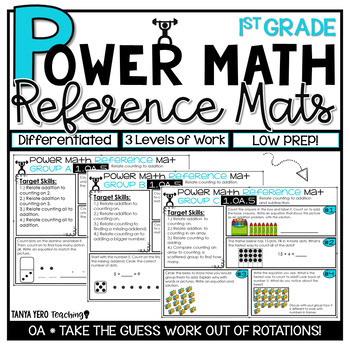# 1st Grade Guided Math Rotations | Algebraic Thinking | Differentiated WorkSubjects
Standards
Resource Type
Formats Included
• PDF
Pages
100 pages
##### Also included in
1. Take the prep work out of guided math rotations and make life easier for yourself. POWER Math Reference Mats tailor instruction for your students with NO PREP on your behalf! Differentiate math with ease! What are Guided Math Reference Mats?POWER Math Reference Mats are designed to assist teachers
\$22.00
\$28.96
Save \$6.96
2. The POWER Math Ultimate Bundle is everything you need for a successful year of math instruction! The resources found in this bundle were designed with the philosophy in mind that math should be POWERful. POWER stands for purposeful opportunities with engagement and rigor. You and your students deser
\$64.00
\$92.94
Save \$28.94

### Description

Take the prep work out of guided math rotations and make life easier for yourself. POWER Math Reference Mats tailor instruction for your students with NO PREP on your behalf! Differentiate math with ease!

What are Guided Math Reference Mats?

POWER Math Reference Mats are designed to assist teachers during math rotations. Housed with specific target skills for each level in your class, POWER Math Reference Mats come with various problems for you to give to your students when working in small groups. Group A is for students who are in need of remediation, Group B is for students working on grade level, and Group C is for students who need enrichment. Each mat comes with target skills that are designed to match the needs of each student level in your class. There are questions/problems for each target skill which can be given to your students. Power Math Reference Mats require little materials: whiteboards, expo markers, and erasers or paper and pencils.

~~~~~~~~~~~~~~~~~~~~~~~~~~~~~~~~~~~~~~~~~~~~~~~~~~~~

Standards & Topics Covered

Operations and Algebraic Thinking

➥ 1.OA.1 – Use addition and subtraction within 20 to solve word problems

➥ 1.OA.2 – Solve word problems that call for addition of three whole numbers whose sum is less than or equal to 20

➥ 1.OA.3 – Apply properties of operations as strategies to add and subtract

➥ 1.OA.4 – Understand subtraction as an unknown-addend problem

➥ 1.OA.5 – Relate counting to addition and subtraction

➥ 1.OA.6 – Add and subtract within 20, demonstrating fluency for addition and subtraction within 10

➥ 1.OA.7 – Understand the meaning of the equal sign

➥ 1.OA.8 – Determine the unknown whole number in an addition or subtraction equation

~~~~~~~~~~~~~~~~~~~~~~~~~~~~~~~~~~~~~~~~~~~~~~~~~~~~

Total Pages
100 pages
Included
Teaching Duration
1 month
Report this Resource to TpT
Reported resources will be reviewed by our team. Report this resource to let us know if this resource violates TpT’s content guidelines.

### Standards

to see state-specific standards (only available in the US).
Use addition and subtraction within 20 to solve word problems involving situations of adding to, taking from, putting together, taking apart, and comparing, with unknowns in all positions, e.g., by using objects, drawings, and equations with a symbol for the unknown number to represent the problem.
Solve word problems that call for addition of three whole numbers whose sum is less than or equal to 20, e.g., by using objects, drawings, and equations with a symbol for the unknown number to represent the problem.
Apply properties of operations as strategies to add and subtract. If 8 + 3 = 11 is known, then 3 + 8 = 11 is also known. (Commutative property of addition.) To add 2 + 6 + 4, the second two numbers can be added to make a ten, so 2 + 6 + 4 = 2 + 10 = 12. (Associative property of addition.)
Understand subtraction as an unknown-addend problem. For example, subtract 10 â 8 by finding the number that makes 10 when added to 8.
Relate counting to addition and subtraction (e.g., by counting on 2 to add 2).